Taxicab Geometry
 What is Taxicab Geometry? T-Line Segment T-Polygon T-Line T-Circle T-Ellipse Outlook Taxicab Geometry on the Internet References .
 To the Main Page   "Mathematische Basteleien"

What is Taxicab Geometry?
 ...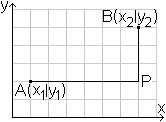... Taxicab geometry is a form of geometry, where the distance between two points A and B is not the length of the line segment AB as in the Euclidean geometry, but the sum of the absolute differences of their coordinates.  Formula:  AP+PB= |x2-x1| + |y2-y1| The points have only integer coordinates in the simple version and their connections are grid lines.

 ...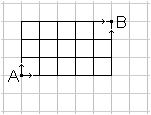... These are the following facts behind the name.  You can consider the grid as a net of streets, which a taxi driver negotiates.  The crossings are the places, where he can stop.  It is remarkable that the taxi driver - starting in A - can take different ways, which have the same length, if he is continuously approaching the destination B.
If the taxi driver stays on one line of the grid, there is no difference to the usual geometry.

There are also the names  City-Block-, Manhattan- oder Minkowski-Geometrie beside Taxicab Geometry.
Hermann Minkowski (1864 to 1909) had the idea to this kind of geometry.

The example of this web page is a chapter in Martin Gardner's book (1).

T-Line Segment   top
There is one line segment to one length in Euclidean geometry, but several line segments to one length in taxicab geometry.
 ...... This is again shown by the example on the left. Both lines have the length 9.  You call this new kind of line taxicab-line or t-line.

 ...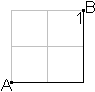... ...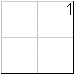... There is the question, how many t-line segments are between two points.  You can count them in this simple case. There are 6 of them.

 ...... Looking at the number of t-lines systematically, you discover a law.  The number of t-line segments on a crossing is always the sum of the segments at the foregoing crossings. This leads to Pascal's triangle, whose rows are diagonal here.

 ...... ...... Point B is on the ninth diagonal and thus on the ninth row of  Pascal's  triangle, related to A, and on the fourth place.  There is the number 28+56=84 or "9 choose 3". There are 84 t-lines for the case "6 to the right, 3 to the top" to go from A to B.  Generally the number is "n+m choose m" for the case "m to the right, n to the top".

T-Line
 ...... For the sake of completeness here is any line between A and B, which is not a t-line segment.  It consists of the four t-line segments AC, CD, DE and EB.

T-Polygon    top
You must also look at polygons in a new way.
 ...... Here are two t-line segments of the length 8 between the points A and B. You get a 2-gon, which doesn't exist in the Euclidean geometry.
If you give a third point C, it is a question of definition, whether the points A, B, and C form a triangle.
>Pro: They form a triangle in the Euclidean geometry.
>Against: A, B, and C lie on a t-line.

In this sense there is also the t-quadrilateral ADBC in this figure with the side length 4.
Consequently the figure is also a t-16-gon of the side length 1, though strange enough.

T-Squares?
 ...... You can go a step forward and call the t-quadrilateral ADBC a t-rhombus, because four sides are equal. It is not a t-square, because the angle at point D is 180°. There is a problem in this definition. Many features of the rhombus get lost, for example the symmetry.

A quadrilateral is a square, when all sides are equal und all angles 90° in Euclidean geometry.

 ...... In this sense the quadrilaterals on the left are t-squares. If you connect the points by straight lines, you get a rhombus. Thus is it a t-rhombus and no t-square?

 ...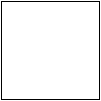... If you demand all features of the square, you must only take the square of the Euclidean geometry as t-square.

Another access to the square gives the equation |x|+|y|=2 with D=|R. You can transfer it to taxicab geometry easily.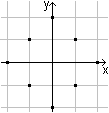You will experience on this page that this t-square is also a t-circle. This is strange.

T-Line    top
 ...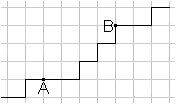... You get a t-line from a t-line segment, if you elongate the t-line segment over both ends.  You must mind that it goes always upwards (here) or downwards.

 ...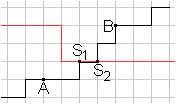... If you look for points of intersections between two t-lines, you can have each number of points.  Here the two t-lines intersect in two points. If you change the ascending (black) t-line in the way that it also goes horizontally starting at S1, then there are points of intersection without limits.
It is not worth to work on t-lines, because there are so many.
The t-circle and the t-ellipse in the next chapters are more interesting.

T-Circle    top
One circle
 ...... If you give one fixed point M and draw all points, which have the same distance r from the centre M, you get a circle.

This statement is transferred  to taxicab geometry.
 ...... If you give one fixed point M and draw all points, which have the same t-distance 3 from the centre, you get 12 points. They form a square of the t-side length 6 standing on one vertex. More generally: If you give one fixed point M and draw all points, which have the same t-distance r from the centre M, you get 4r points. They form a square of the t-side length 2r standing on one vertex.

This reminds one to squaring the circle, where you must look for a square with the same area as has a circle.

If the circle is centered at the origin (0, 0), then the equation is  x²+y²=r² in the Cartesian coordinate system.
In taxicab geometry there is |x|+|y|=r.

 ...Maybe you shouldn't connect the points of the t-circle. The impression of being a square is lost.

Two circles
 ...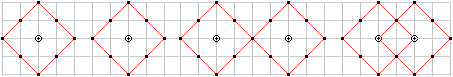... Two equal t-circles intersect in all, no one, in one, or in two points like the usual circles.

There are more cases with t-circles.
 ...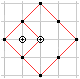... Two t-circles with the radii R and r can intersect in more than two points, for instance in three points here.  In this corner position the number of the points is determined by the smaller t-radius.  It is n=2r+1 for r

T-Ellipse   top
Horizontal position
 ...... If you give two fixed point F1 und F2 and draw all points, which have the same sum of the distances from  them, you get an ellipse. The sum is s=s1+s2 in the drawing. The two points F1 und F2 are called foci (singular form is focus).

The distance b of the foci and the constant sum s determine the shape of the t-ellipse like the usual ellipse.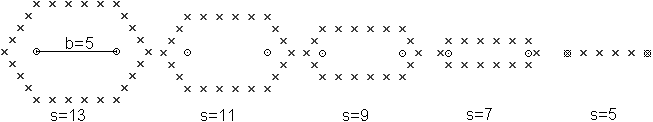A simple computer program finds out that figures of two trapezia arise. One pair of parallel sides is as long as the line segment b=F1F2 . There is only one row in the case s=b=6 with the foci at the ends.here: b=2, s=6 The computer only found t-ellipses, when either  > b and s are even like in the drawing on the left, or  >b and s are odd like in the computer drawings above.

Explanation: For the left angular point there is the equation  s=2x+b or s-b=2x or "s-b is an even number".
Thus both, s and b, must be even or odd at the same time.

Diagonal position
You receive other figures, if the foci lie diagonally.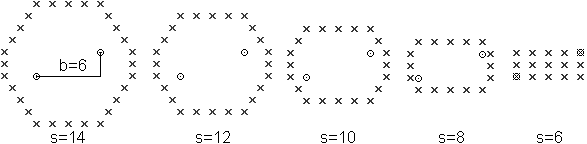A simple computer program finds out that figures arise, which have a rectangle 3x5 as a base. There is the complete rectangle 3x5 in the case s=b with the foci at the vertices.

How to get a t-ellipse with b=6 and s=101 The point top right lies on a t-line segment through the foci. There is (2+6)+2=10.
2 You find five more points with other t-line segments in the same way.
3 There are six more points for symmetry reasons.
4 There are four more points at the top. The distance to the right focus is 1 greater step by step, and 1 smaller to the left  focus. - The same thoughts explain the five points at the bottom.
5 You must transfer the same explanation to the points lying vertically. Here you get two more points, on the left and on the right.
You can generalize these steps to all t-ellipses.

 ...... So it results in the fact that the numbers of the horizontal and vertical points are determined by the points of the slope triangle. These are the numbers 5 and 3.  Above, the foci lie horizontally. There the two numbers are 1 and 5, alternatively 1 and 3.

 ......are The computer program found out that a complete rectangle 3x5 is also a t-ellipse. It is b=s=6. If the foci are diagonal in the centres of grid squares as on the left, the result is the same rectangle 3x5, but with b=s=8.

Outlook top
>There are also investigations about the t-hyperbola und der t-parabola in Gardner's book. Both curves can also be identified by distances.

>You can also take triangle patterns instead of square patterns.

>You can also give a three-dimensional or a higher-dimensional grid to do taxicab geometry.

Taxicab Geometry on the Internet       top

German

English

Barile, Margherita (MathWorld)
Taxicab Metric

Jim Wilson
Overview of Taxi Cab Geometry

Robert M. Dickau
Shortest-path diagrams3D shortest-path diagramsPaths through a 4-D Lattice

The Wolfram Demonstrations Project
Taxicab Geometry

References   top
(1) Martin Gardner: Geometrie mit Taxis, die Köpfe der Hydra und andere mathematische Spielereien, Basel 1997
[ISBN 3-7643-5702-9]
Martin Gardner: The Last Recreations: Hydras, Eggs, and Other Mathematical Mystifications

Feedback: Email address on my main page